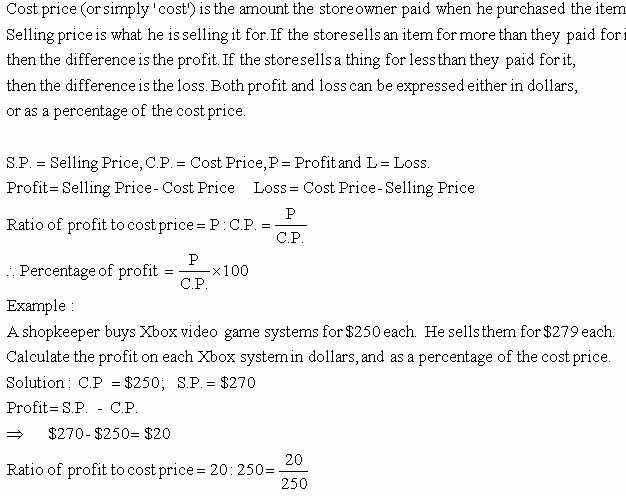Email us to get an instant 20% discount on highly effective K-12 Math & English kwizNET Programs!

#### Online Quiz (WorksheetABCD)

Questions Per Quiz = 2 4 6 8 10

### MEAP Preparation - Grade 7 Mathematics1.111 Finding Profit PercentagePercentage of profit = (20/250) x 100 = 0.08 = 8% Directions: Answer the following questions. Also write at least ten examples of your own.
 Q 1: Cost price = \$6000 and selling price = \$8000, what is the profit percentage?33 1/3%5 1/33%1 3/33% Q 2: Cost price = \$2500 and selling price = \$3000, what is the profit percentage?25%20%15% Q 3: Cost price = \$5500 and selling price = \$7000, what is the profit percentage?11 3/27%3 11/27%27 3/11% Q 4: Cost price = \$5000 and selling price = \$6000, what is the profit percentage?30%15%20% Q 5: Cost price = \$4000 and selling price = \$4500, what is the profit percentage?1 2/12%12 1/2%2 1/12% Q 6: Cost price = \$3600 and selling price = \$4000, what is the profit percentage?1 9/11%9 1/11%11 1/9% Q 7: Cost price = \$290 and selling price = \$300, what is the profit percentage?13 3/29%3 13/29%29 3/13% Q 8: Cost price = \$3000 and selling price = \$3300, what is the profit percentage?30%15%10% Question 9: This question is available to subscribers only! Question 10: This question is available to subscribers only!